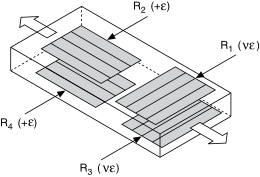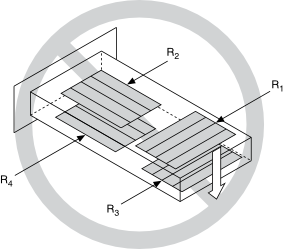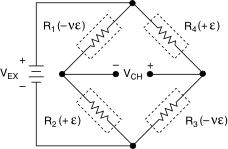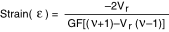# Full-Bridge Type III

## NI-DAQmx 18.6 Help

Edition Date: January 2019
Part Number: 370466AH-01
View Product InfoNI-DAQmx 18.6 HelpNI-DAQmx 19.0 HelpNI-DAQmx 19.1 HelpNI-DAQmx 19.5 Help

The following figure shows how to position strain gage resistors in an axial configuration for the full-bridge type III.The full-bridge type III configuration only measures the axial configuration.Full-bridge type III strain gage configurations have the following characteristics:
• Four active strain gage elements. Two are mounted in the direction of axial strain with one on the top side of the strain specimen and the other on the bottom side. The other two act together as a Poisson gage and are mounted transverse, or perpendicular, to the principal axis of strain with one on the top side of the strain specimen and the other on the bottom side.
• Compensation for temperature.
• Rejection of bending strain.
• Compensation for the aggregate effect on the principle strain measurement due to the Poisson's ratio of the material.
• Sensitivity at 1000 µε is ~ 1.3 mVout / VEX input.
Related concepts

## Full-Bridge Type III Circuit DiagramThe following symbols apply to the circuit diagram:
• R1 is the active strain gage element measuring compressive Poisson effect (–ε).
• R2 is the active strain gage element measuring tensile strain (+ε).
• R3 is the active strain gage element measuring compressive Poisson effect (–ε).
• R4 is the active strain gage element measuring the tensile strain (+ε).
• VEX is the excitation voltage.
• RL is the lead resistance.
• VCH is the measured voltage.

The following equation converts voltage ratios to strain units for full-bridge type III configurations.where Vr is the voltage ratio that virtual channels use in the voltage-to-strain conversion equation, GF is the gage factor, and v is the Poisson's ratio.